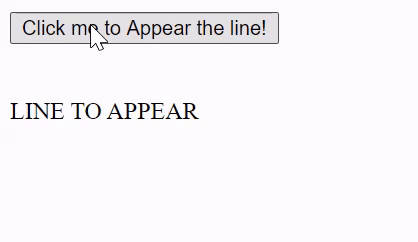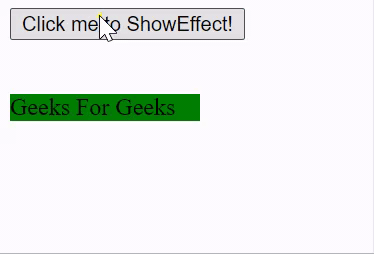Related Articles

# script.aculo.us Appear Effect

• Last Updated : 23 Nov, 2020

In this article, we will demonstrate the effect of Appear by using a JavaScript library called script.aculo.us. The appear effect offers a smooth appearing transition to the element. We can also adjust the duration of this effect as well.

Syntax:

`Effect.Appear('element_id');`

// or

`Effect.Appear('element_id', [options]);`

Options:

• duration: Duration take to fade the element, defaults value is 1.0.
• from: It is a float value representing the percent of opacity to start, the default value is 0.0.
• to: It is a float value representing the percent of opacity to end, the default value is 1.0.

Note: To use this library, we are supposed to install the library and then use it in our programs. And to do so you can follow this link http://script.aculo.us/downloads.

Example 1: To demonstrate the use of this function, we have written a small piece of code. In which we have written a small JavaScript function named ShowEffect method which uses the Appear method of this library. By clicking on Click me to Appear the line! , you will see the effect clearly.

To see the effect, first install the library and then open this program in the local environment.

## HTML

 ```<``html``>`` ` `<``head``>``    ``<``script` `type``=``"text/javascript"` `        ``src``=``"prototype.js"``>``    ````     ` `    ``<``script` `type``=``"text/javascript"` `        ``src``=``"scriptaculous.js?load = effects,controls"``>``    ````     ` `    ``<``script` `type``=``"text/javascript"``>``        ``function ShowEffect(element) {``            ``new Effect.Appear(element, ``            ``{ duration: 1, from: 0, to: 1.0 });``        ``}``    `````` ` `<``body``>``    ``<``div` `onclick``=``"ShowEffect('geeks_1')"``>``        ``<``button` `type``=``"button"``>``            ``Click me to Appear the line!``        ````    ````    ``<``br` `/>``    ``<``br` `/>`` ` `    ``<``div` `id``=``"geeks_1"``>``        ``LINE TO APPEAR``    `````` ` ``

Output:Example 2:

## HTML

 ```<``html``>`` ` `<``head``>``    ``<``script` `type``=``"text/javascript"` `        ``src``=``"prototype.js"``>``    ````     ` `    ``<``script` `type``=``"text/javascript"` `        ``src``=``"scriptaculous.js?load = effects,controls"``>``    ````     ` `    ``<``script` `type``=``"text/javascript"``>``        ``function ShowEffect(element) {``            ``new Effect.Appear(element, ``            ``{ duration: 1, from: 0, to: 1.0 });``        ``}``    `````` ` `<``body``>``    ``<``div` `onclick``=``"ShowEffect('geeks_1')"``>``        ``<``button` `type``=``"button"``>``            ``Click me to Appear the line!``        ````    ````    ``<``br` `/>``    ``<``br` `/>`` ` `    ``<``div` `id``=``"geeks_1"``>``        ``<``div` `style="width: 10%; height: 10%; ``            ``background-color: green;">``            ``Geeks For Geeks``        ````    `````` ` ``

Output:My Personal Notes arrow_drop_up